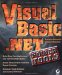# Summary

 Team Fly

Page 622

### Summary

In this chapter we discussed some advanced graphics topics by means of two demonstration applications: a practical application for plotting 2-dimensional functions and a ''fun" application that generates fractals. While building the plotting application you learned how to build GraphicsPath objects and how to apply transformations to graphics elements before rendering them in the drawing surface. You also learned how to calculate arbitrary math expressions at runtime with the MSScript ActiveX control.

The second sample application of this chapter was a simple fractal generator that produces startling fractal images. This application generates the fractals by painting one pixel at a time. The calculation of each pixel's color involves some math, which isn't beyond the grasp of the average developer. You can enhance the fractal generator in many ways and the most challenging aspect of the application is the design of a palette for coloring the fractals.

 Team FlyVisual Basic .NET Power Tools
ISBN: 0782142427
EAN: 2147483647
Year: 2003
Pages: 178

Similar book on Amazon